### Detailed Caption for Median Filtering Illustration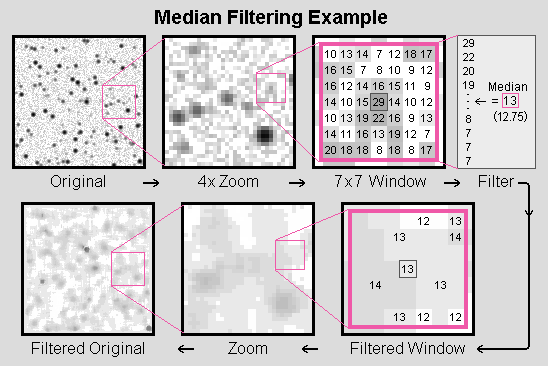Move clockwise starting in the upper-left corner...

The original is a 128×128 pixel synthetic starfield generated by a program which uses a 2-D Gaussian function to make the simulated stars. In this example, a FWHM (Full Width at Half Maximum) of 1.67 pixels was used to set the stars' size. Each pixel was broken down into a 3×3 sub-pixel array for star generation. 200 stars were placed at random locations. The fainter stars tend to be contained within approximately 3×3 pixel areas, while the brightest stars can be seen occupying a 5×5 pixel area. Thus, at least about 10% of the pixels belong to stars in this example.

A probabilistic exponential function was used to distribute the stars' brightnesses across the available range (such that there are more faint stars than bright stars), though about a third of the stars are too faint to be seen in a casual visual inspection of the original. This is because the generating program also allows the addition of Gaussian noise to the image, which was done in this case, thus obliterating the faintest stars in the subsequent background noise. Note that Gaussian noise is not the same as the signal-dependent Poisson noise which would be present in the star images in a real astronomical frame (along with some other sources of noise which would be more or less Gaussian), though it still can be illustrative of what happens at the sky level.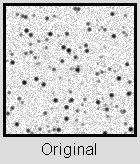The image generating program uses 16 bits for the image data, but the range in the actual data used here turned out to be -64 to 21,120, which is more like a 14.37 bit range. This is less than 16 bits because: 1) the maximum pixel value depends on the star brightness generating function; and 2) how close the brightest star is to being exactly centered on a particular pixel -- both randomized events -- and the program maintains a little headroom to prevent overflows in a best case (worst case?) scenario. The image minimum is less than zero because of the added noise, which can be either positive or negative.

The number of bits in the data was subsequently reduced by a linear transformation to 12 bits (0 to 4095 range) for the example shown here which was actually processed by the median filter. The median pixel value for the entire image is found to be 12.81, which is taken as the blank sky background level. This value is known to be too high by less than 0.5 because of the presence of the stars in the image. Synthesized images can be very useful for developing and testing software algorithms because you know what answer(s) you should get.

For display only, each image was further transformed to 8 bits (256 shades of grey from white to black). This was done with a logarithmic function. This greatly expands the contrast at the background level (making faint detail more visible, but also exaggerating the noise) while compressing the contrast at the bright (black) end of the scale. Thus, the displayed images look much more like photographic negatives (which are also logarithmic) than they would be otherwise. The transform algorithm also "sets-to-white" any pixel which has a data value lower than a level of 0.32·sigma below the image median, which means that the lower 75% of the pixels whose values are negative with respect to the background level are zeroed out for the display.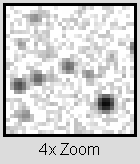In this example, data values of 11 and below go to zero, while data value 12 has the first step above white (13 goes to 22 steps above white, while 14 goes to the 35th step -- showing how sharp the contrast increase is at the background level). This "clipping" of the below-background pixels has the effect of slightly minimizing the apparent granular noise seen due to the addition of the Gaussian noise and exaggerated by the logarithmic transformation's increase in contrast at the background level. Note that these transformations only apply to the images displayed here, not to the "data" which was run through the median filter.

The middle frame shows a four times enlargement of the 32×32 pixel area inside the pink box on the original. This region was chosen because it happens to have a relatively wide range of star brightnesses within it, which is beneficial for purposes of illustration. In particular, this region contains a fairly faint, though still distinct, star which serves as a good example of the filter's effect near the limit of detection. Other such areas in the original could have been chosen -- this was simply one of the first which caught my eye and so I went with it for the rest of the example. It is not the result of any detailed hunting around in the original to find an optimum example to focus on.

The 7×7 window frame shows the area outlined in pink on the 4x enlargement, along with the actual values of all the pixels within the window. These are the values which are operated on by the median filter at this location in the image. Recall that the background level is about 13 and the maximum possible pixel value is 4095. The window size was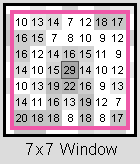chosen to contain approximately twice as many pixels as the brighter stars (which, one recalls, tend to be contained within 5×5 areas). With about 10% of the pixels belonging to stars, the typical 7×7 window contains one star. With a less crowded field one could use a larger filtering window before reaching this condition.

The window shown is centered on the brightest pixel of the faint star. Comparison of the pixel values in the immediate vicinity of the center pixel shows that this particular star seems to be centered horizontally fairly well on the center pixel, but is not well-centered vertically. In fact, it can be seen that the star's center is towards the bottom of its center pixel. The sum of the data values in a 3×4 pixel box around this point is 208, which is 54.28 above the background level of 12.81. The average (or mean) for the 49 pixel values in the 7×7 window is 13.59 ±4.39 (s.d), so the star is approximately a 3½ · sigma signal ( s.d. × Sqrt(12) = 15.2 ; 54.3 ÷ 15.2 = 3.57 ), which is about the level at which detection becomes difficult.

The righthand, highlighted box shows the filtering operator acting on the pixel values in the window. At left, the pixel values are ranked from highest down to lowest. The middle value in the list is 13, which is the filter's output for this location in the image, meaning the original pixel value of 29 is replaced by 13. (The computational algorithm doesn't quite actually rank-sort the pixel values to determine the median in this manner, but the effect is the same.)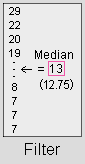A more sophisticated analysis of the distribution of the pixel values (numerically, not spatially) allows a non-integral value of 12.75±¼ to be determined (the value in parentheses), which is right in line with the whole-image median value of 12.81. Thus, this particular window turns out to be rather typical of the entire image in this regard. While there's no reason in principle why the non-integral value for the median couldn't be used, the program doesn't.

Note that the average pixel value in the window of 13.59 is almost one whole data value higher than the median. This is certainly due primarily to the presence of the star in the window, as can be seen by comparing the sum of the pixels containing the star (54.3) to the number of pixels in the window (49): 54.3 ÷ 49 = 1 (approximately).

The lower row of images simply show the median filtered versions of the same areas of the original seen in the top row, with the sequence ending at lower left with the full frame view. The faint star is effectively removed by the filter, but brighter stars are not. Increasing the window size would have caused even the brightest stars to be filtered out of the resultant image.

Because of the way the contrast at the background level is increased for the display frames, what passes through the filter is greatly exaggerated. The maximum pixel value has dropped from 21,120 in the original to only 150 in the median filtered image. The minimum goes from -64 up to +10, the latter value only being a couple of counts below the sky level. Therefore, it can be instructive to view the difference image between the original and the filtered data: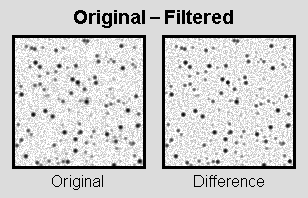If the difference image looks a little bit sharper than the original image, this is because subtracting off a median image from an original is one type of unsharp masking -- a common image sharpening technique. In this particular example, a slightly larger window (9×9 or 11×11) might have led to somewhat more sharpening by increasing the low-pass filtering properties of the median filter.